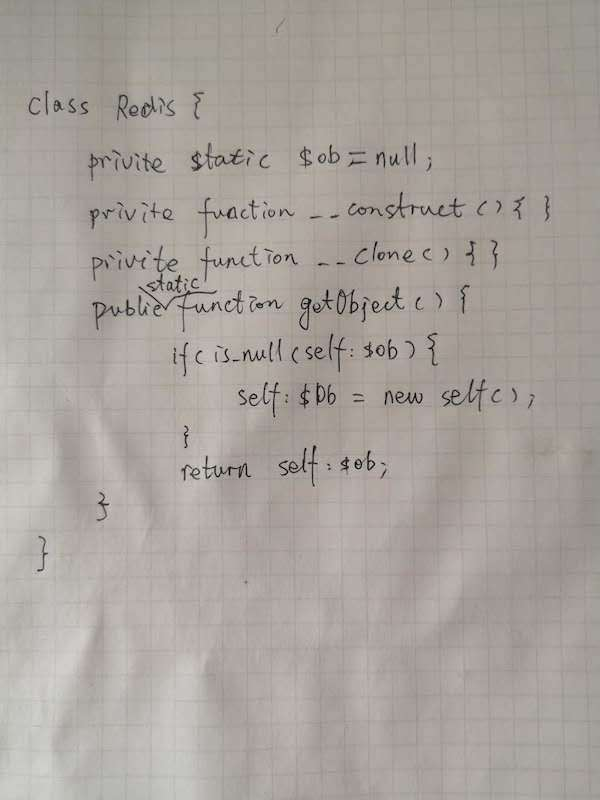# week3 命题作业## 手写单例﻿

## 设计模式-组合模式代码

interface Component{    public function __construct($name); public function add(Component$c);    public function run($dipth);} 叶子实现 class Leaf implements Component{ private$name;    public function __construct($name) {$this->name = $name; } public function add(Component$c)    {        echo "叶子不能添加子节点";    }    public function run($dipth) { echo str_repeat('-',$dipth) . $this->name . PHP_EOL; }} 组件实现 class Composite implements Component{ private$name;    private $list = []; public function __construct($name)    {        $this->name =$name;    }    public function add(Component $c) {$this->list[] = $c; } public function run($dipth)    {        echo str_repeat('-', $dipth) .$this->name . PHP_EOL;        foreach ($this->list as$component) {            $component->run($dipth + 2);        }    }}

class Client{    public function run()    {        $root = new Composite('window form');$root->add(new Leaf('picture'));        $root->add(new Leaf('button'));$root->add(new Leaf('button'));        $frame = new Composite('frame');$frame->add(new Leaf('label'));        $frame->add(new Leaf('checkbox'));$frame->add(new Leaf('textbox'));        $frame->add(new Leaf('label'));$frame->add(new Leaf('label'));        $frame->add(new Leaf('linklabel'));$frame->add(new Leaf('passwordbox'));        $root->add($frame);        \$root->run(1);    }}(new Client())->run();

-window form---picture---button---button---frame-----label-----checkbox-----textbox-----label-----label-----linklabel-----passwordbox﻿

﻿## 评论